# Algebraic Properties of Real Numbers

The basic algebraic properties of real numbers a,b and c are:

1. Closure: a + b and ab are real numbers
2. Commutative: a + b = b + a, ab = ba
3. Associative: (a+b) + c = a + (b+c), (ab)c = a(bc)
4. Distributive: (a+b)c = ac+bc
5. Identity: a+0 = 0+a = a
6. Inverse: a + (-a) = 0, a(1/a) = 1
7. Cancelation: If a+x=a+y, then x=y
8. Zero-factor: a0 = 0a = 0
9. Negation: -(-a) = a, (-a)b= a(-b) = -(ab), (-a)(-b) = ab

 More combination rules
Index

 HyperPhysics****HyperMath*****Algebra R Nave
Go Back

# Algebraic Combinations

Factors with a common denominator can be expanded: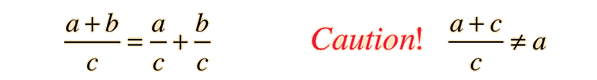Fractions can be added by finding a common denominator: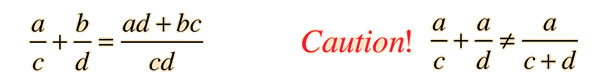Products of fractions can be carried out directly: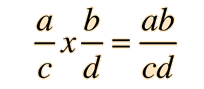Quotients of fractions can be evaluated by inverting and multiplying: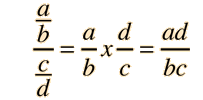Index

 HyperPhysics****HyperMath*****Algebra R Nave
Go Back

# Algebraic Equations

The role of a basic algebraic equation is to provide a formal mathematical statement of a logical problem. A first order algebraic equation should have one unknown quantity and other terms which are known. The task of solving an algebraic equation is to isolate the unknown quantity on one side of the equation to evaluate it numerically. Using x as the unknown and other letters to represent known quantities, consider the following example equation: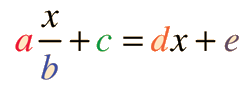The strategy for solving this equation is the repeated application of the golden rule of algebra to collect like terms and isolate the quantity x on one side of the equation.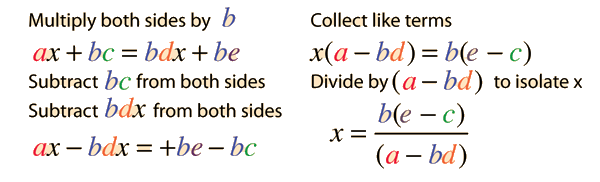Practice Equation
Index

 HyperPhysics****HyperMath*****Algebra R Nave
Go Back

# Algebra Practice Equation

Solving a basic algebraic equation involves repeated applications of the golden rule of algebra to isolate the unknown quantity on one side of the equation. Using x as the unknown and other letters to represent known quantities, consider the following example equation: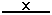+ =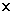+

Try your hand at this calculation. You should get=Note that there are a number of circumstances where a solution does not exist. If b=0, then the first term is infinite, so the calculation defaults to the value b=1 if no value of b is entered in order to avoid this condition for an infinity. Any set of values for which a=bd will also give an infinity, as can be seen from the expression for x above.

Index

 HyperPhysics****HyperMath*****Algebra R Nave
Go Back

# Golden Rule of Algebra

## Any mathematical operation can be performed on one side of an equation so long as the identical operation is performed on the other side of the equation.

The solution of basic algebraic equations is accomplished by applying this rule repeatedly in order to isolate the unknown quantity and evaluate it. The application of this rule - proceeding by doing clear symmetric operations on the two sides of an equation - can help avoid most common mistakes in solving algebraic equations.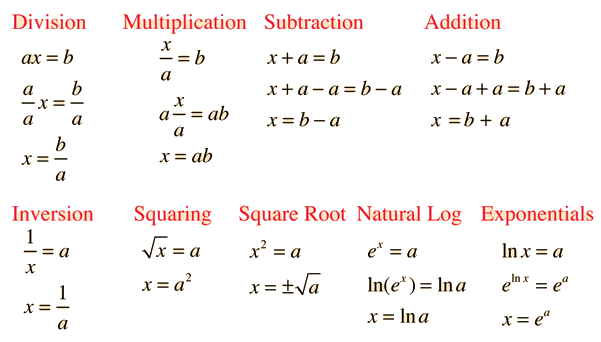Index

 HyperPhysics****HyperMath*****Algebra R Nave
Go Back